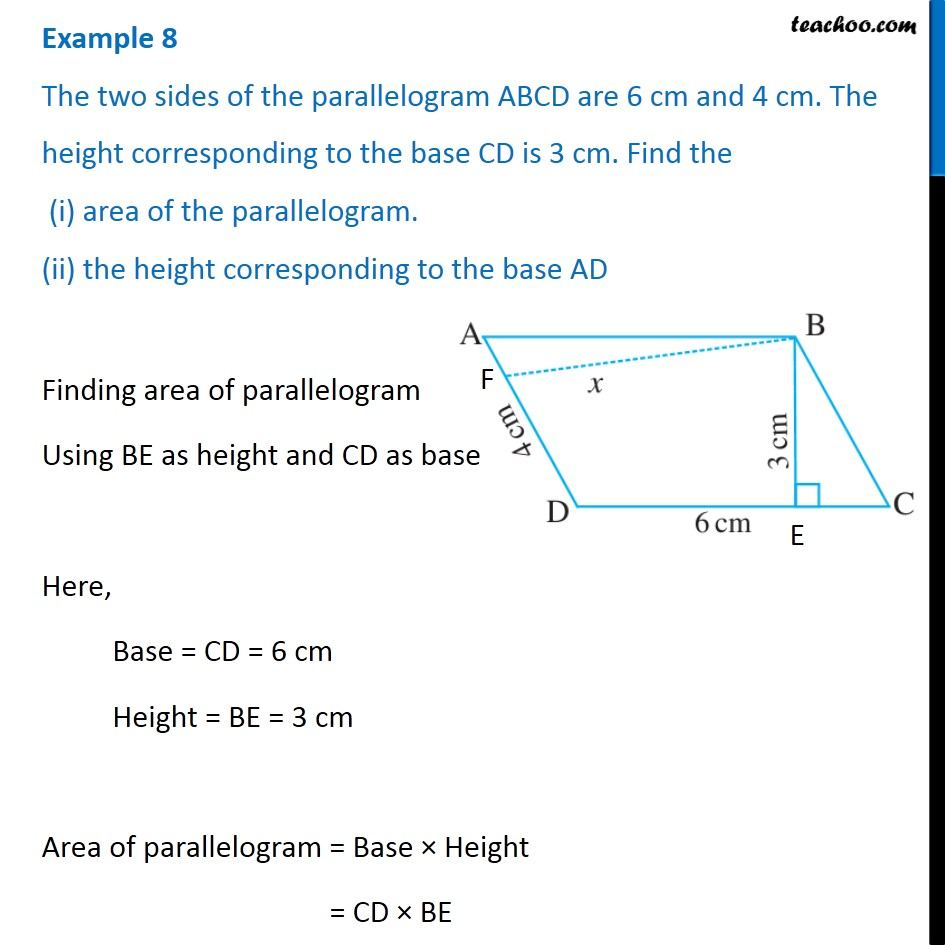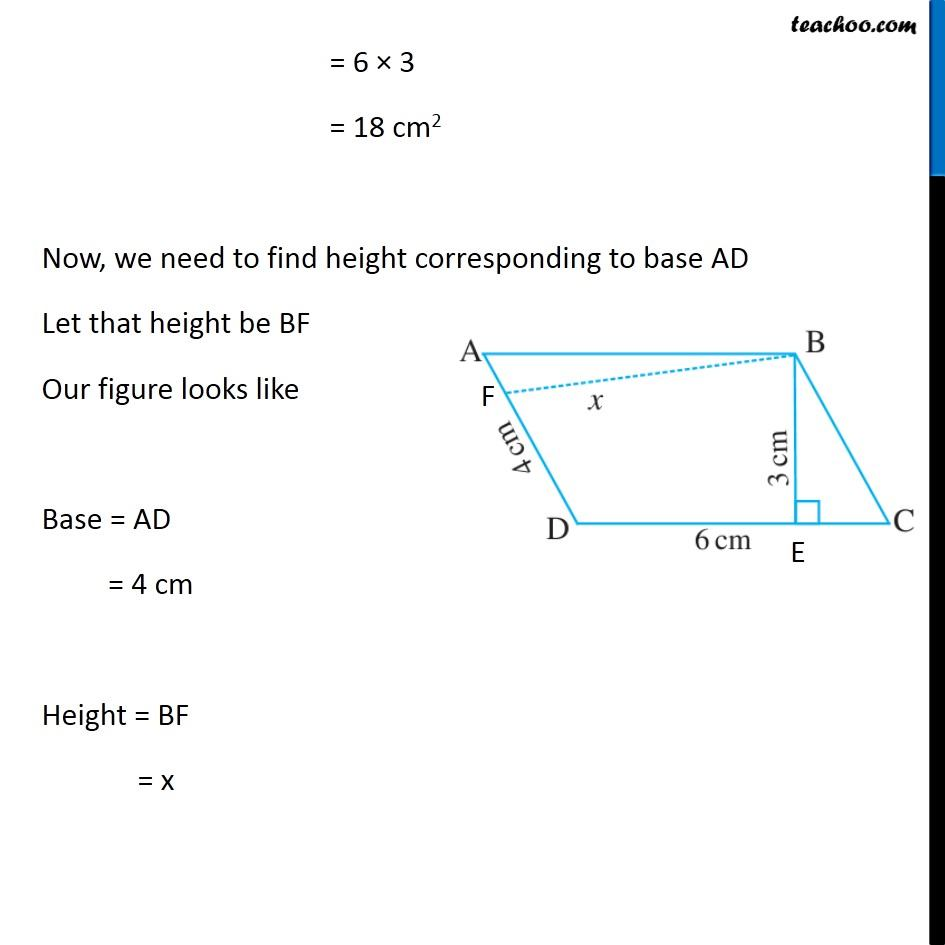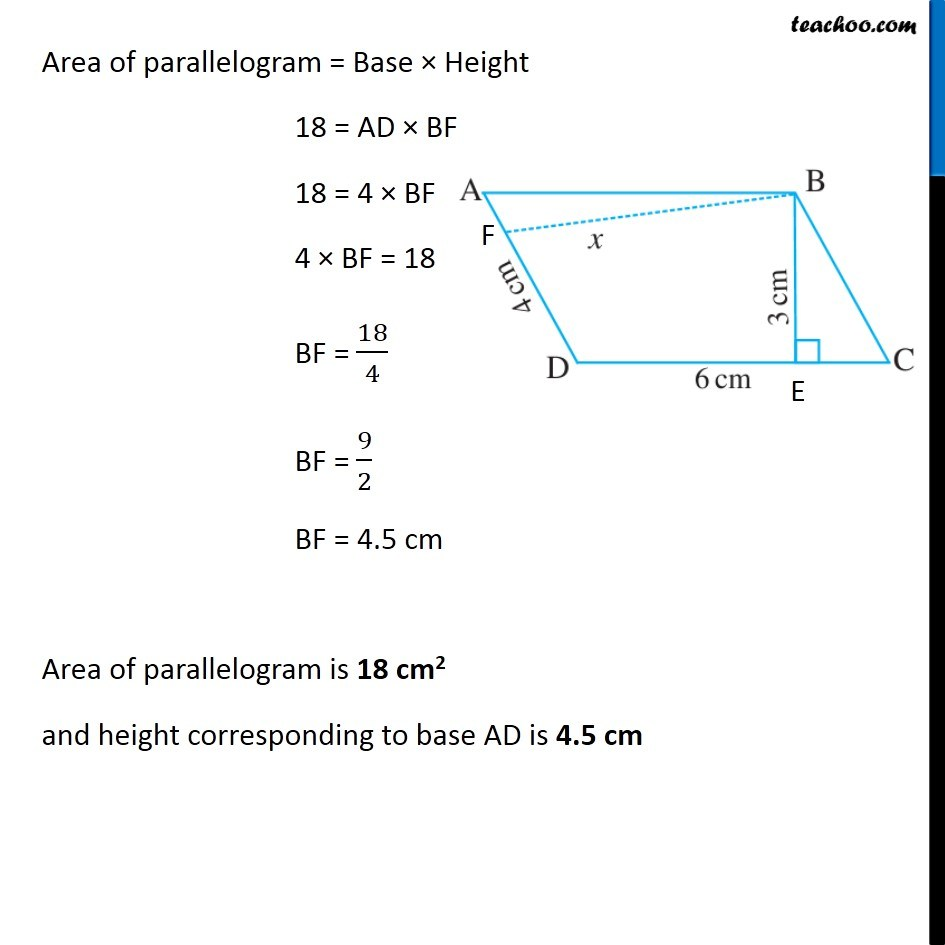Area of parallelogram

Chapter 9 Class 7 Perimeter and Area
Concept wiseGet live Maths 1-on-1 Classs - Class 6 to 12

### Transcript

Example 3 The two sides of the parallelogram ABCD are 6 cm and 4 cm. The height corresponding to the base CD is 3 cm. Find the (i) area of the parallelogram. (ii) the height corresponding to the base AD Finding area of parallelogram Using BE as height and CD as base Here, Base = CD = 6 cm Height = BE = 3 cm Area of parallelogram = Base × Height = CD × BE = 6 × 3 = 18 cm2 Now, we need to find height corresponding to base AD Let that height be BF Our figure looks like Base = AD = 4 cm Height = BF = x Area of parallelogram = Base × Height 18 = AD × BF 18 = 4 × BF 4 × BF = 18 BF = 18/4 BF = 9/2 BF = 4.5 cm Area of parallelogram is 18 cm2 and height corresponding to base AD is 4.5 cm